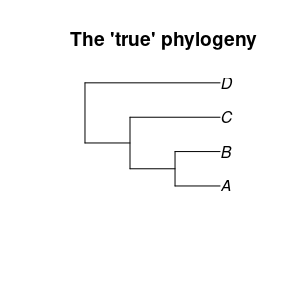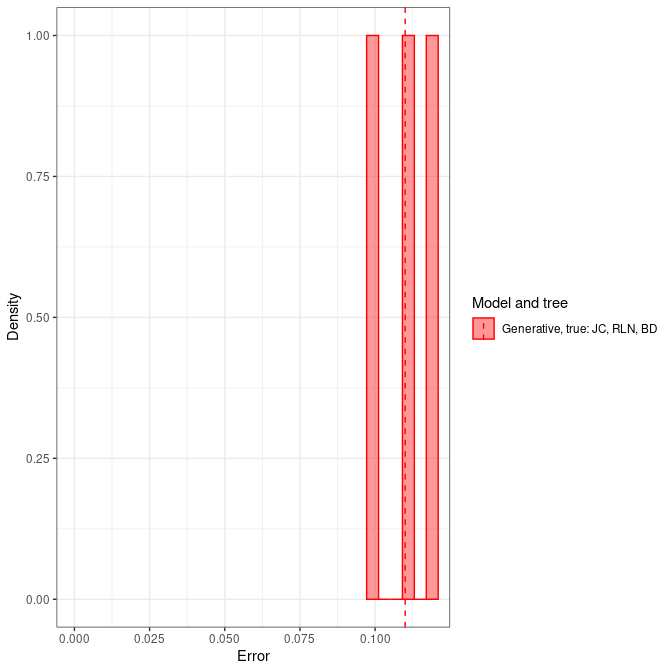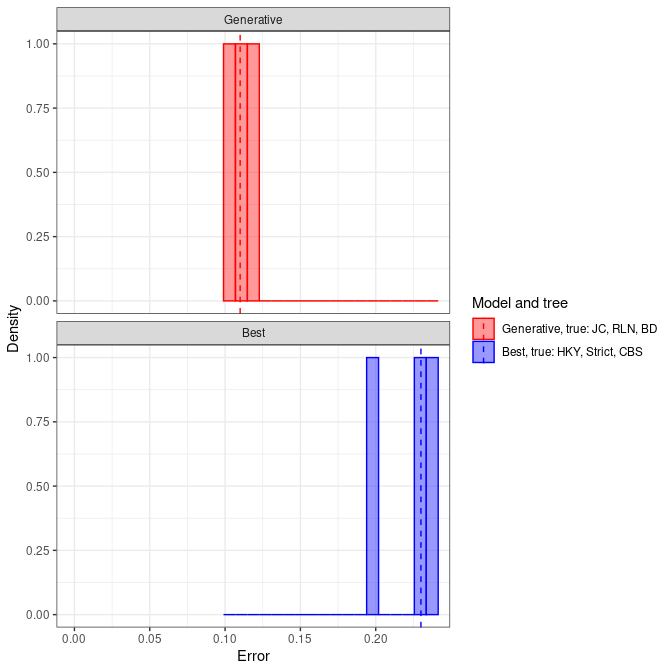# pirouette demo

#### 2023-01-19

The goal of pirouette is to estimate the error BEAST2 makes from a known phylogeny. This phylogeny can be created using a non-BEAST speciation model, for example the PBD or MBD models.

library(pirouette)

pirouette has a the option to do model comparison, picking the model that has most evidence (aka marginal likelihood). This option only works under Linux and Mac. When working on such a system, the BEAST2 package ‘NS’ is expected to be installed.

In this demo, we use a simple ‘true’ phylogeny:

true_phylogeny <- ape::read.tree(text = "(((A:1, B:1):1, C:2):1, D:3);")
ape::plot.phylo(true_phylogeny, main = "The 'true' phylogeny")As this vignette is a demo (and not a thorough research), we’ll use a short alignment:

alignment_params <- create_alignment_params(
root_sequence = create_blocked_dna(length = 20)
)

## 1. Only use the generative model in inference

There are multiple ways to select for an inference model. In the simplest case, which is the default, we will do our inference with the generative model only:

type run_if measure evidence inference model
generative always FALSE Default
experiment <- create_test_gen_experiment()
experiments <- list(experiment)

Here we measure the baseline error: the error that BEAST2 makes when the inference model is the correct generative model (JC69 and strict clock):

pir_params <- create_pir_params(
alignment_params = alignment_params,
experiments = experiments
)

Running it:

errors <- NULL

if (beastier::is_beast2_installed()) {
errors <- pir_run(
phylogeny = true_phylogeny,
pir_params = pir_params
)
} else {
errors <- create_test_pir_run_output(
)
}

Here we show the errors as a table:

knitr::kable(utils::head(errors))
tree inference_model inference_model_weight site_model clock_model tree_prior error_1 error_2 error_3
true generative 0.5 JC69 relaxed_log_normal birth_death 0.1 0.11 0.12

Here we plot the error:

pir_plot(errors)## 2. Do inference on candidate models

Again, there are multiple ways to select for which inference model(s) to use. Here we assume we do not know the generative model. Instead, we only run the inference model that has the highest evidence (also called ‘marginal likelihood’).

type run_if measure evidence inference model
candidate best_candidate TRUE Yule
candidate best_candidate TRUE Birth-Death
if (rappdirs::app_dir()$os != "win") { experiment_yule <- create_test_cand_experiment( inference_model = create_test_inference_model( tree_prior = create_yule_tree_prior() ) ) experiment_bd <- create_test_cand_experiment( inference_model = create_test_inference_model( tree_prior = create_bd_tree_prior() ) ) # Use the same files to work on, as only one will actually run an experiment experiment_bd$beast2_options <- experiment_yule$beast2_options experiment_bd$inference_model$mcmc <- experiment_yule$inference_model$mcmc experiment_bd$errors_filename <- experiment_yuleerrors_filename experiments <- list(experiment_yule, experiment_bd) check_experiments(experiments) pir_params <- create_pir_params( alignment_params = alignment_params, experiments = experiments, evidence_filename = get_temp_evidence_filename() ) } Note that we select the model with the heighest evidence from a set of just one model (JC69, strict, Yule). Run this setup: if (rappdirs::app_dir()os != "win" &&
is_beast2_installed() &&
is_beast2_ns_pkg_installed()
) {
errors <- pir_run(
phylogeny = true_phylogeny,
pir_params = pir_params
)
} else {
}

Here we show the errors as a table:

knitr::kable(utils::head(errors))
tree inference_model inference_model_weight site_model clock_model tree_prior error_1 error_2 error_3
true generative 0.5 JC69 relaxed_log_normal birth_death 0.1 0.11 0.12
true candidate 0.4 HKY strict coalescent_bayesian_skyline 0.2 0.23 0.24

Here we plot the error:

pir_plot(errors)## 3. Do inference with the generative and model with most evidence

Again, there are multiple ways to select for which inference model(s) to use. Next to the known generative model (JC69 and strict clock), here we use the inference model that has the highest evidence (also called ‘marginal likelihood’).

Here we measure the baseline error, from the generative model, and the error that BEAST2 makes when the inference model is the one with most evidence:

type run_if measure evidence inference model
generative always FALSE Yule
candidate best_candidate TRUE Birth-Death
experiment_yule <- create_test_gen_experiment(
inference_model = create_test_inference_model(
tree_prior = create_yule_tree_prior()
)
)
if (rappdirs::app_dir()os != "win") { experiment_bd <- create_test_cand_experiment( inference_model = create_test_inference_model( tree_prior = create_bd_tree_prior() ) ) experiments <- list(experiment_yule, experiment_bd) pir_params <- create_pir_params( alignment_params = create_test_alignment_params(), experiments = experiments, evidence_filename = get_temp_evidence_filename() ) } else { experiments <- list(experiment_yule) pir_params <- create_pir_params( alignment_params = create_test_alignment_params(), experiments = experiments ) } Note that we select the model with the heighest evidence from a set of just one model (JC69, strict, Yule). Run this setup: if (rappdirs::app_dir()os != "win" &&
is_beast2_installed() &&
is_beast2_ns_pkg_installed()
) {
errors <- pir_run(
phylogeny = true_phylogeny,
pir_params = pir_params
)
} else {
}

Here we show the errors as a table:

knitr::kable(utils::head(errors))
tree inference_model inference_model_weight site_model clock_model tree_prior error_1 error_2 error_3
true generative 0.5 JC69 relaxed_log_normal birth_death 0.1 0.11 0.12
true candidate 0.4 HKY strict coalescent_bayesian_skyline 0.2 0.23 0.24

Here we plot the error:

pir_plot(errors)